﻿ 一种贵州CPI影响因素动态关系分析方法 A Technique of Dynamic Relationship Analysis of Influencing Factors for Guizhou CPI

Advances in Applied Mathematics
Vol. 08  No. 03 ( 2019 ), Article ID: 29143 , 9 pages
10.12677/AAM.2019.83049

A Technique of Dynamic Relationship Analysis of Influencing Factors for Guizhou CPI

Minzhen Wu1, Youfu Wu2*

1Guizhou Minzu University, Guiyang Guizhou

2Guizhou Jiaotong College, Guiyang Guizhou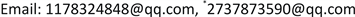Received: Feb. 14th, 2019; accepted: Feb. 27th, 2019; published: Mar. 6th, 2019ABSTRACT

Based on the relevant economic theory and the previous research system of influencing factors of CPI, in this paper, the qualitative theory was used to select the macroeconomic indicators as candidate indicators for influencing CPI macroeconomic factors in Guizhou. Then, the quantitative analysis method was used to select the main macroeconomic factors influencing CPI fluctuation for Guizhou. The vector autoregressive model was established with these factors and CPI. The impulse response function and variance decomposition were used to analyze the main external factors of CPI in Guizhou. The results show that CPI is impacted more quickly than itself, followed by the commodity retail price index, while other factors also have a certain impact on the fluctuation of CPI.

Keywords:Influencing Factor Analysis, VAR Model, Impulse Response Analysis, Variance Decomposition

1贵州民族大学，贵州 贵阳

2贵州交通职业技术学院，贵州 贵阳Copyright © 2019 by author(s) and Hans Publishers Inc.1. 引言

1) 在分析CPI的波动会受到哪些经济指标影响时，对经济指标的选取往往是通过经济学理论和主观经验意识来选取，这样将会遗漏一些影响相对较小但又不可忽视的经济指标。

2) 在选择分析CPI范围上，分析较多的是某一国家或某一较发达区域的CPI，然而对较为落后区域的CPI分析相对较少，尤其是对贵州CPI的分析更是少之又少。

2. 影响因素指标的选取

2.1. 候选指标的选取Table 1. Candidate Indicators and Detailed description

2.2. 数据说明

2.3. CPI影响因素选取方法Table 2. MIC Coefficient of CPI and Candidate Indicators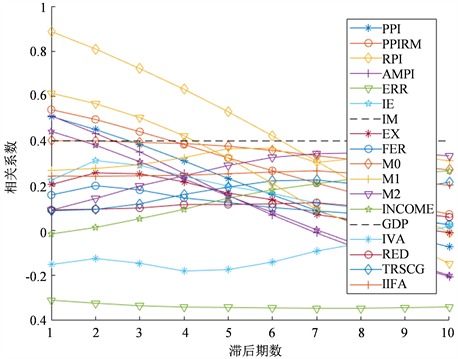Figure 1. Pearson correlation coefficient map with lag of 10 periods

3. 影响因素动态分析

3.1. 平稳性检验

3.2. 建立VAR模型

$DCPI=\left(\begin{array}{l}-0.354\\ 0.0421\\ -0.007\\ -0.053\\ 0.6255\\ 0.0368\\ 0.0071\end{array}\right)\left(\begin{array}{l}DCPI\left(t-1\right)\\ DFER\left(t-1\right)\\ DAMPI\left(t-1\right)\\ DIIFA\left(t-1\right)\\ DRPI\left(t-1\right)\\ PPIRM\left(t-1\right)\\ TRSCG\left(t-1\right)\end{array}\right)+\left(\begin{array}{l}0.1883\\ 0.0198\\ -0.021\\ 0.0456\\ -0.185\\ -0.042\\ -0.011\end{array}\right)\left(\begin{array}{l}DCPI\left(t-2\right)\\ DFER\left(t-2\right)\\ DAMPI\left(t-2\right)\\ DIIFA\left(t-2\right)\\ DRPI\left(t-2\right)\\ PPIRM\left(t-2\right)\\ TRSCG\left(t-2\right)\end{array}\right)+1.028C$ (1)Figure 2. VAR Model stability test

3.3. 脉冲响应分析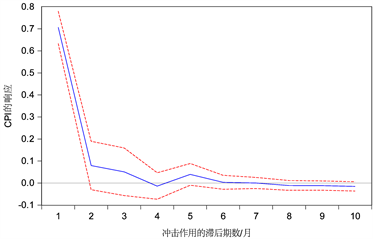(a)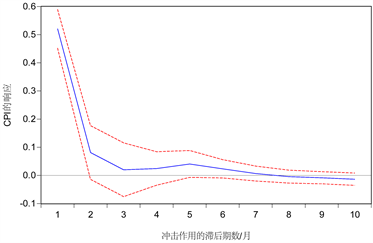(b)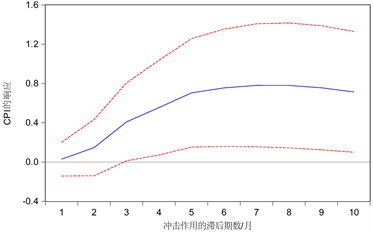(c)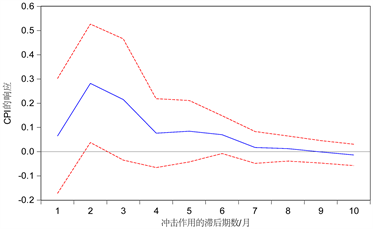(d)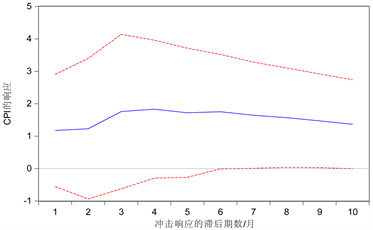(e)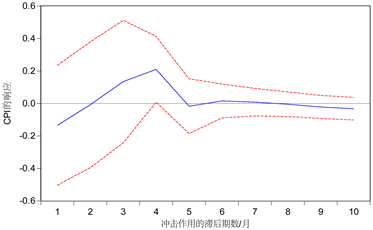(f)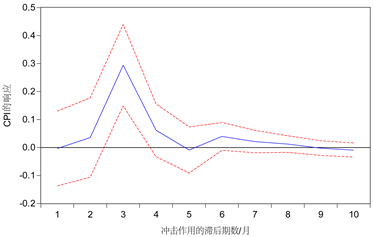(g)

Figure 3. (a). Response function of CPI shock to itself; (b) Response Function of RPI Shock to CPI; (c) Response Function of PPIRM Shock to CPI; (d) Response Function of AMPI Shock to CPI; (e) Response Function of TRSCG Shock to CPI; (f) Response Function of FER Shock to CPI; (g) Response Function of IIFA Shock to CPI

3.4. 方差分解Table 6. Contribution rate of variance decomposition (%)

4. 结论与建议

A Technique of Dynamic Relationship Analysis of Influencing Factors for Guizhou CPI[J]. 应用数学进展, 2019, 08(03): 430-438. https://doi.org/10.12677/AAM.2019.83049

1. 1. Ahmed, E.M. and Suliman, Z. (2011) The Long-Run Relationship between Money Supply, Real GDP, and Price Level: Empirical Evidence from Sudan. Journal of Business Studies Quarterly, 2, 68-79.

2. 2. Ding, Z., Zhou, M. and Ning, B. (2011) Research on the Influencing Effect of Coal Price Fluctuation on CPI of China. Energy Procedia, 5, 1508-1513. https://doi.org/10.1016/j.egypro.2011.03.258

3. 3. 谭智慧. 基于函数型数据分析的CPI影响因素分析[D]: [硕士学位论文]. 天津: 天津大学, 2012.

4. 4. 郑兆顺. 基于多元回归模型和逐步分析法的濮阳市CPI影响因素分析[J]. 濮阳职业技术学院学报, 2017, 30(1): 80-84.

5. 5. 易丹辉. 时间序列分析: 方法与应用[M]. 北京: 中国人民大学出版社, 2011.

NOTES

*通讯作者。